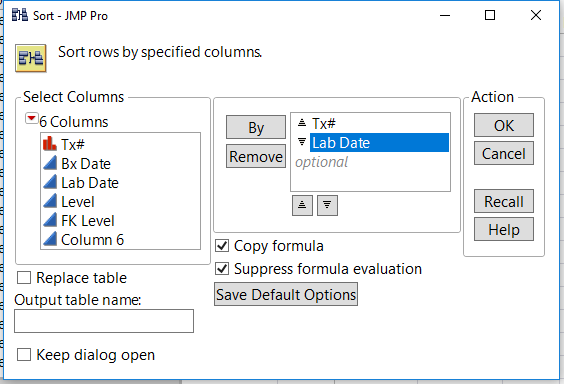Choose Language Hide Translation Bar
Highlighted

## formula help!

I am using  JMP® Pro 14.2.0

I am not very experienced at writing JMP formulas. What I am trying to do is compare average medication levels for patients who have had a biopsy. The biopsies happen at irregular time intervals, therefore, most of the cells in the Bx Date column are blank (no biopsy happened at that date, but they were tested for their medication level). If I could write a formula to label the cells within 3 months of a given Bx Date I can then using that column to filter out only the data that is within 3 months of a biopsy date and start doing some statistics. Ideally the formula would also first make sure the drug levels are coming from the same patient (Tx# refer to individual patients).

thanks so much for your help

6 REPLIES 6
Highlighted

## Re: formula help!

Here is one way to do it.  It first finds all of the Biopsy dates within the range of the current Lab Date, and then finds the biopsy closest to the current Lab Date from the found Biopsys

``````dt = Current Data Table();
x = .;
Tx = :Tx#;
theRows = dt << get rows where( Tx == :Tx# & :Bx Date >= :Lab Date - In Days( 90 ) & :Bx Date <= :Lab Date + In Days( 90 ) );
If( N Rows( theRows ) > 0,
If( N Rows( theRows ) == 1,
x = :Bx Date[theRows],
x = :Bx Date[theRows];
For( i = 2, i <= N Rows( theRows ), i++,
If( Abs( :Bx Date[theRows[i]] - :Lab Date ) < Abs( x - :Lab Date ),
x = :Bx Date[theRows[i]]
)
);
)
);
x;``````
Jim
Highlighted

## Re: formula help!

Thanks for your fast reply. If I apply your formula I get the following…. (See screenshot). Unfortunately the dates in the new “column 6” go past the Bx Date…..thus is not ideal since my question is how do the medication level leading up to the biopsy effect the biopsy result? Is there a way to tweak this so that at the time of the biopsy the date in column 6 will change to the date of the next biopsy from the Bx Date column?

Thanks, sorry to bother you with this.

Highlighted

## Re: formula help!

The key to the formula, is the selection of which Biopsy dates to look at.  If you don't want to examine any Biopsy date after the current Lab Date, then all one has to do, is to change the selection criteria

``````dt = Current Data Table();
x = .;
Tx = :Tx#;
theRows = dt << get rows where( Tx == :Tx# & :Bx Date >= :Lab Date - In Days( 90 ) & :Bx Date <= :Lab Date  );
If( N Rows( theRows ) > 0,
If( N Rows( theRows ) == 1,
x = :Bx Date[theRows],
x = :Bx Date[theRows];
For( i = 2, i <= N Rows( theRows ), i++,
If( Abs( :Bx Date[theRows[i]] - :Lab Date ) < Abs( x - :Lab Date ),
x = :Bx Date[theRows[i]]
)
);
)
);
x;``````
Jim
Highlighted

## Re: formula help!

Hi @cjw0 ,

Given your restated criteria, I think this formula becomes much more straight forward if you sort the table by ascending Tx# and descending Lab Date:Then create a new formula column with the following:

``````If(
Row() == 1 | :Lab Date == :Bx Date | :Tx# != Lag( :Tx# ), :Bx Date,
Is Missing( :Bx Date ) & Lag( :Column 6 ) - :Lab Date <= In Days( 90 ), Lag( :Column 6 )
)``````

Hope this helps.

Highlighted

## Re: formula help!

This email and/or any documents in this transmission is intended for the addressee(s) only and may contain legally privileged or confidential information. Any unauthorized use, disclosure, distribution, copying or dissemination is strictly prohibited. If you receive this transmission in error, please notify the sender immediately and return the original.

Ce courriel et tout document dans cette transmission est destiné à la personne ou aux personnes à qui il est adressé. Il peut contenir des informations privilégiées ou confidentielles. Toute utilisation, divulgation, distribution, copie, ou diffusion non autorisée est strictement défendue. Si vous n'êtes pas le destinataire de ce message, veuillez en informer l'expéditeur immédiatement et lui remettre l'original.
HighlightedRyan_GilmoreCommunity Manager

## Re: formula help!

Here is a slightly different approach than @txnelson . Rather than populate the column with date values, the formula indicates if the lab date is within 3 months of the biopsy date. It uses this approach since some of the lab dates fell within 3 months of more than one biopsy date.

``````dt = Current Data Table();
currentPatientId = :Tx#;
latestBxDates = :Bx Date[dt << get rows where(
!Is Missing( :Bx Date ) & currentPatientId == :Tx#
)];
nDays = In Days( 90 );
Any( :Lab Date - nDays < latestBxDates < :Lab Date + nDays );``````
Article Labels

There are no labels assigned to this post.